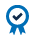##### Education Home

Option traders have many different definitions of skew. In probability theory and statistics, skew is a measure of the asymmetry of the probability distribution of a real-valued random variable about its mean.1

On a practical level, traders quote skew in terms of price or volatility. One common measure of skew is the difference in implied volatility of two options – one put, one call – with the same absolute value of delta or same moneyness. This is known as the risk reversal. Traders use the risk reversal to express a view of the cost of downside protection versus upside protection. For example, the 25-delta risk reversal is calculated by finding both the 25-delta call and put and then taking the difference between the implied volatilities of those two specific options.

Looking at this implied volatility curve, the put has vol of 70 while the call has vol of 45.  Therefore, the risk reversal is calculated as negative 25 vol points. This curve would be ‘skewed’ to the downside.

Just as simple variance uses all the available option prices for volatility as opposed to a single at-the-money option, CVOL skew calculations use all the available option prices on each side of the at-the-money rather than just two individual options.

In the more traditional method, the 25-delta put is representative of the left-hand side of the area under the curve and the downside of the market.  Similarly, the 25-delta call represents the right side of the curve and the upside of the market.

The traditional risk reversal method of calculating skew uses only two points on the implied volatility curve and therefore misses much of the available market information.

CVOL skew uses all the puts on the left side to represent the downside and all the calls on right side of the curve to represent the upside.

CVOL skew can be broken into two components: down variance and up variance, referred to as DnVar and UpVar.

DnVar is calculated by doubling the put area and using the same calculation that determines CVOL. In this example, the calculated put sigma is 68.

Similarly, UpVar is calculated by doubling the call area and using the same calculation that determines CVOL. In this example, the calculated call sigma is 45, which happens to be the same as the risk reversal call. Adjustments need to be made around at-the-money to ensure proper redistribution of variance for calls and puts.

In this example, UpVar is 45, DnVar is 68.

The difference of -23 vol points and the ratio is .6618. By using all the available strikes, we get a more precise calculation of skew.

DnVar, UpVar, and skew are part of the CVOL family of indexes. The CVOL Index provides a data set calculated using CME Group’s methodology on its own options prices. Live Streaming indices are available on CME Direct and supporting independent service vendors (ISV), and historical data is available through CME Datamine and Quickstrike.  These calculations are made daily, updated at the end of every trading day, and stored in a downloadable format.

CVOL Indexes, from CME Group, utilize the simple variance estimate method to provide expected volatility metrics derived from the entire implied volatility curve.

 Source: Wikipedia

#### ACCREDITED COURSEIn case you didn’t know, the CFA Institute allows its members to self-determine and report continuing education credits earned from external sources. CFA Institute members are encouraged to self-document such credits in their online CE tracker. CME Institute offers a variety of courses, webinars, and white papers to support your professional education.